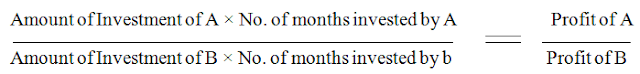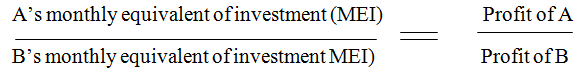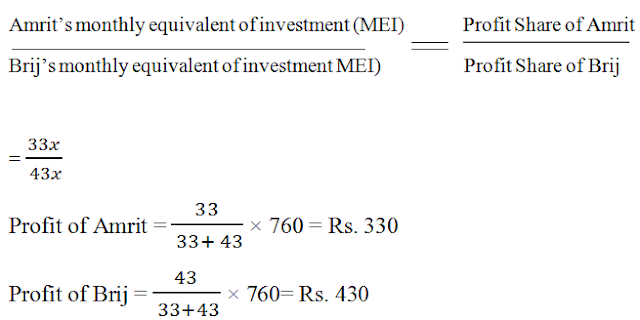# Partnership Questions with Tricks

Today, I am sharing the 'Partnership' chapter from quantitative aptitude. It is also an important chapter because 1-2 questions in exams mostly comes from this chapter. Partnership chapter with examples is given below. I hope it will be beneficial for you.

### Meaning and Introduction

#### Definition of 'Partnership' as per Partnership Act, 1932

"Partnership is relation between persons who have agreed share the profits of a business carried on by all any of them acting for all."

### Formulae

Following are some formulae to solve the questions based on this chapter. Learn these formulas carefully .

### If the business is run by Two Partners

Suppose A and B are two partners that run the business. In such a case following formula is used:Another formula is(MEI stands for Monthly Equivalent Investment)

#### Example 1.

Akshay and Bobby together invested Rs. 12,000 in a business. At the end of the year, out of a total profit of Rs. 1800, Akshay's share was Rs. 750. What was the investment of Akshay?
As from the above information, profits are shared in the ratio of their investents. Money invested by A and B for the same period so, following formula will be apply.### If the business is run by more than Two Partners

In such a case, following formula is applied.

MEI of A : MEI of B : MEI of C = Profit of A : Profit of B : Profit  of C

#### Example 2.

Ajay, Bashir and Cherman enter into a partnership. Ajay contributes Rs. 320 for 4 months, Bashir contributes Rs. 510 for 3 moths and Cherman contributes Rs. 270 for 5 months. If the total profit is Rs. 208, find the profit share of the partners.
MEI of Ajay : MEI of Bashir : MEI of Cherman= Ajay's profit : Bashir's Profit : Cherman's Profit
(MEI stands for Monthly Equivalent Investment)
= (320 × 4) : (510 ×  3) : (270 ×  5)
= 1280 : 1530 : 1350
= 128 : 153 :135### When Amount of Capital Invested is not given

Lets take the example to understand this case.

#### Example 3.

Amrit and Brij entered into partnership with capitals in the ratio of 4:5. After 3 months, Amrit withdrew 1/4th of his capital and B withdrew 1/5th of his capital. The gain at the end of 10 months was Rs. 760. Find their shares of profits.
As the ratio of capitals of Amrit and Brij are given 4 : 5
Let, the capitals of Amrit and Brij be 4x and 5x respectively. Hence, monthly equivalent of investment  (MEI) of Amrit
=Similarly, monthly equivalent of investment of Brij is:As we know:### Some Other Type of Questions

#### Example 4.

What amount of money is divided between Ashok, Bindusara and Chandragutpa if Bindusara and Chandragupta together get Rs. 100 and Ashok gets twice as much as Bindusara while Chandragupta with Ashok gets Rs. 150?
(For ease of the solution take Ashok,Bindusara and Chandragupta as A, B and C)
Given that
B + C = 100
A + C = 150
A = 2B
2B + C = 150
B + (B + C) = 150    [Since B + C = 100]
B = 150 - 100 = 50
A + B + C = (A + C) + B = 150 + 50 = Rs. 200

#### Example 5.

In a partnership, A invests 1/6 of the capital for 1/6 of the time, B invests 1/3 of the capital for 1/3 of the time and C, the rest of the capital for whole time. Find A's share of the total profit of Rs. 2300.
Capital of C = 1 - 1/6 - 1/3 = 1/2
Let the total time be 12 months
A's profit : B's profit : C's profit = MEI of A : MEI of B : MEI of CShare of Ashok = 1/ 23 × 2300 = Rs. 100
So, above are the some formulas to understand the 'Partnership' chapter. So, Keep the practice and All the Best.